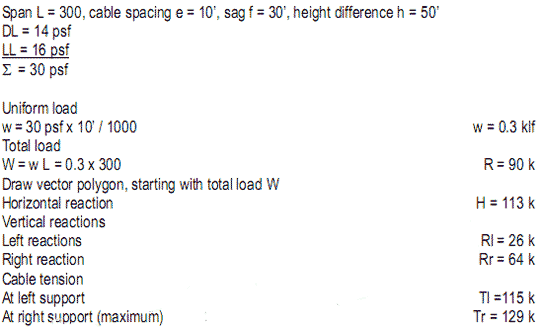## Wednesday, January 29, 2014

### STRUCTURES: FORCE VS. STRESS

Force and stress refer to the same phenomena, but with different meanings.  Force is an external action, measured in absolute units: # (pound), k (kip); or SI units: N (Newton),  kN (kilo Newton).  Stress is an internal reaction in relative units (force/area ), measured in psi (pound per square inch), ksi (kip per square  inch); or SI units: Pa (Pascal), kPa (kilo Pascal).  Axial stress is computed as:

f = P / A

where

f = stress
P = force
A = cross section area

Note: stress can be compared to allowable stress of a given material.

Force is the load or action on a member
•  Stress can be compared to allowable stress for any material, expressed as:
F ≥ f  (Allowable stress must be equal or greater than actual stress)

where

F = allowable stress
f = actual stress

The type of stress is usually defined by subscript:

Fa, fa   (axial stress, capital F = allowable stress)
Fb, fb   (bending stress, capital F = allowable stress)
Fv, fv   (shear stress, capital F = allowable stress)

The following examples of axial stress demonstrate force and stress relations:

Note:  The heel would sink into the wood, yield it and mark an indentation

## Wednesday, January 22, 2014

### STRUCTURES - FORCE TYPES

Forces on structures include tension, compression, shear, bending, and torsion.  Their  effects and notations are tabulated below and all but bending and related shear are  described on the following pages.  Bending and related shear are more complex and further described in the next part.

1  Axial force (tension and compression)
2 Shear
3 Bending
4 Torsion
5 Force actions
6  Symbols and notations

A Tension
B Compression
C Shear
D Bending
E Torsion

## Monday, January 13, 2014

### STRUCTURES - SUSPENSION ROOF

Assume:1  Cable roof structure

2  Parabolic cable by graphic method

Process:
Draw AB and AC (tangents of cable at supports)
Divide tangents AB and AC into equal segments
Lines connecting AB to AC define parabolic cable envelop

3 Cable profile

Process:
Define desired cable sag f (usually f = L/10)
Define point A at 2f below midpoint of line BC
AB and AC are tangents of parabolic cable at supports
Compute total load W = w L

4  Equilibrium vector polygon at supports (force scale: 1” = 50 k)
Process:
Draw vertical vector (total load W)
Draw equilibrium polygon W-Tl-Tr
Draw equilibrium polygons at left support Tl-H-Rl
Draw equilibrium polygons at right support Tr-Rr-H
Measure vectors H, Rl, Rr at force scale

Note:  This powerful method finds five unknowns: H, RI, Rr. Tl. Tr The maximum cable force is at the highest support

## Sunday, January 5, 2014

### DESIGN FUNICULAR STRUCTURES

Graphic vector are powerful means to design funicular structures, like arches and  suspension roofs; providing both form and forces under uniform and random loads.

Arch
Assume:

1 Arch structure

2  Parabolic arch by graphic method

Process:
Draw AB and AC (tangents of arch at supports)
Divide tangents AB and AC into equal segments
Lines connecting AB to AC define parabolic arch envelope

3 Arch profile

Process:
Define desired arch rise D (usually D = L/5)
Define point A at 2D above supports
AB and AC are tangents of parabolic arch at supports
Compute vertical reactions R = w L /2

4  Equilibrium vector polygon at supports (force scale: 1” = 50 k)  Process:

Draw vertical vector (reaction R)
Complete vector polygon (diagonal vector parallel to tangent)
Measure vectors (H = horizontal reaction, F = max. arch force)

Note:

The arch force varies from minimum at crown (equal to horizontal reaction), gradually increasing with arch slope, to maximum at the supports.